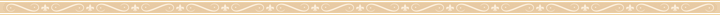Marco Fumagalli

The calculation of the lots, according to Placidus.

(Linguaggio Astrale 103, June 1996), english translation by Daria Dudziak

According to the system of Placidus, the lots may be defined as the points of the local space chart which, having the same declination as the point B, are as many degrees away from it in right ascension (RA), as the point C is away from a point A in oblique ascension (OA) and in the same direction. The points A and B may be planets, house cusps or even lots; the point C is usually the oriental horizon or horoscope (hor). The name and the astrological meaning of the lot depend on the quality of the points A and B, on the basis of which the lot is calculated: the lot calculated from the Sun to Saturn is called the father's lot as these two celestial bodies are the significators of the father. Two general formulas for the calculation of the lots may be expounded, one which makes possible to find its AR and another its AO:

OA (hor) - OA (A) + RA (B) = RA lot
OA (hor) - OA (A) + OA (B) = OA lot

The two points have to be reversed between them if at the moment we are considering the Sun is under the horizon: for example in order to find the mother's lot in a diurnal figure we take Venus as the point A and the Moon as the point B, but if the figure is nocturnal we do the opposite. On the other hand several lots do not change from the day to the night as, for example, that of marriage. We show as an example the calculation of the Moon's lot, also called Tychê or Part of Fortune and indicated with the following symbol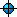:

by day: OA (hor) - OA (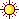) + RA (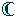) = ARby night: OA (hor) - OA () + RA () = ARThe following two figures should explain the principle on which the calculation of the lunar lot and of all the other lots is based (the small arrow indicates the diurnal motion of the local sphere):As I wanted the drawing to be as clear and simple as possible, I have left out the ecliptic in the figures and I have placed the Sun on the equator, at the autumnal equinox. In the figure 1 we see how the lunar lot is situated at exactly the same point where the Moon would be if by turning the sphere counterclockwise we made the Sun rise at the eastern horizon (figure 2). For this reason it was called "the lunar horoscope" seeing that its distance from the Moon measured in equatorial degrees or in time is equal to the distance between the real horoscope and the Sun (C-A).Therefore it is obvious that, like the horoscope and the house cusps, the lots are the points of the local sphere: they express a relation between two points calculated only in virtue of the daily motion and of the equatorial arches.

Let's see now a complete example of calculation beginning from the data of a diurnal geniture: Milan, the 14th of April 1956, 10 hour and 35 minutes. It culminates in the midheaven 25°03' of Pisces which right ascension, RAMC, is of 355°28'. The oblique ascension of the horoscope, OA(hor), is of 85°28':

 RAMC 355°28' the arc of equator (equal to 1 quadrant) + 90°00' 445°28' - 360°00' OA (hor) 85°28'

The Sun is in Aries at 24°24'49",and the Moon is in Gemini at 7°;07'48", the north latitude is of 0°09'36". Their right ascensions (RA), declinations (decl.) and oblique ascensions (OA) in Milan are indicated below; we cite also the ascensional difference(AD) of the Moon or the difference between its RA and its OA which will be useful for us later:

 RA dec OA AD22°36'29" + 09°28'02" 12°50'59"65°16'47" + 21°40'02" 41°27'36" 23°49'00"

Now we have at our disposal all the elements necessary for the calculation of the position of the lunar lot:

1. first of all we start with finding the right ascension (RA) according to the method we explained for a diurnal geniture :

 OA (hor) 85°28'00" OA- 12°50'59" RA+ 65°16'47" RA137°53'48"

2. afterwards as we know the RA of the lot, we can calculate its distance in right ascension from the nearest meridian (MC o IC) which is called "right distance" (RD) or "meridian distance". From the right ascension of the lot we see immediately that it is under the horizon, in the IV quadrant, between the horoscope and the Imum Coeli (IC), since its RA is higher than the OA(hor) and lower than the right ascension of the Imum Coeli: RA(IC) = RA(MC)+180 = 175°28'. Therefore its right distance RD should be calculated from the Imum Coeli:

 RA (IC) 175°28'00" RA- 137°53'48" RD37°34'12"

3. We proceed now to calculate the temporal hours (TH) of the lot that in this case (diurnal nativity) will be the same of the Moon, seeing that the lot, having the same declination shares the ascensional difference (AD) and the temporal, diurnal (THd) and night-time (THn) hours with the Moon.; therefore the AD of the lot is 23°49'00". Since the AD corresponds to the difference between ascensions (oblique and right) that takes place between the rising and the culmination in the space of a quadrant (equal to a quarter of the whole day, therefore to 6 temporal hours), if we divide by 6 this value we get the quantity of equatorial degrees which added to or subtracted from 15° (equal to an equinoctial hour: 15° x 24 =360°), expresses the value of the single temporal , diurnal and night-time hour. The temporal hours will always be higher than 15° in the hemisphere situated to the north of the equator (positive declination), while they will be lower than 15° in the hemisphere south (negative declination). The declination of the Moon and of the lot is positive therefore the calculation is the following:

 Equinoctial Hour 15°00'00" Equinoctial Hour 15°00'00" 1/6 AD+ 3°58'10" 1/6 AD- 3°58'10" THd18°58'10" THn11°01'50"

4. Now we can find the hourly distance (HD) of the lot, dividing the right distance for the temporal nocturnal hours of the Moon (THn). We use the nocturnal hours since we already know that the lot is under the horizon; if instead it had stayed above we would have calculated the right distance from the Midheaven and we would divided it for the diurnal hours.

 RD37°34'12" THn11°01'50" = HD3h 24m 21s

In this moment the lot can already be inserted in the figure and we can calculate all the aspects that it receives in the world or according to the hourly distances. However we still have to find the ecliptical degree in which the lot should be registered that serves us to know which is the ruler of the lot or the planet that has more dignity on that degree. If we knew the equatorial coordinates of the lot (RA and decl.) we could easily find the ecliptical coordinates (longitude e latitude) by means of the trigonometric formulas of conversion between orthogonal coordinates. Nevertheless, since the lot, like fixed stars , does not have its own motion in the sky, but it is a point of the local space chart, we do not have to take its degree of ecliptical longitude (which is a coordinate of the celestial sphere), but its degree of passage in the local space chart. The degree of passage of a point in a space chart is not other than that degree of the ecliptic that in a datum moment transits to the same hourly distance from the meridian in the same quadrant. That point of the ecliptic that in the IV quadrant will have a hourly distance of 3h24m21s from the IC will be therefore the degree in which we should register the lot. We will see now how to find it.

5. First of all we have to find the oblique ascension (or the discension) of the lot in its own circular chart (OACH-ODHC). Between two consecutive angles of the local space chart there is always a distance of 90° of equator, equal to 6 temporal hours (figure 3) and the OACH or the ODCH expresses the position of a star along this semi-arc . A star placed, for example on the horizon is always 6 hours away from the meridian equal to equinoctial 90° and it still has to cross all his diurnal or nocturnal semi-arc.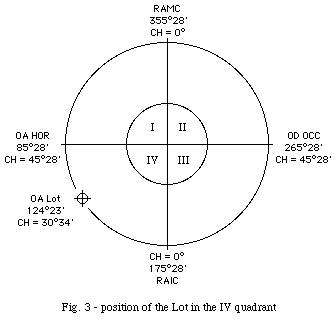In this distance covered the star "consumes" gradually its ascensional difference which is maximum at the horizon (HC = 45°28' in Milan) and reduces to zero on the meridian (CH = 0°), where its OACH-ODCH is equal to its RA since the circualr chart is equal to 0°;. If we add 30° (equal to one HD of 2 hours) to the RA(MC) we get the OACH of the XI house and if we add other 30° to this we find the OAHC of the XII house: this is the principle of the Placidean domification. On the basis of the same principle if we multiply the HD of a star for 15 we get an arc of equator which added to or subtracted from the RA of the nearest meridian make possible for us to find the OAHC or the ODCH of the star, depending on the fact that it is east or west of the meridian. In our example:

 RA (IC) 175°28'00" 15 HD- 51°05'15" OACH124°22'45"

6. Now we have to find the horary circle (CH) or the "pole" of the lot by means of the following formula: tang CH= sin (1/6 ADmax HD) cotang eps, where eps means the obliquity of ecliptic (23°27') and the ADmax means the maximum ascensional difference to a given elevation of the pole (phi). The latter may be found with the following formula: sin ADmax = tang eps tang phi. Since in Milan phi is equal to 45°28', the ADmax is 26°09'41", 1/6 ADmax is equal to 4°;21'37", and the horary circle (CH) of the lot is 30°34'35":

tang CH= sin (4°21'37" 3h24m21s) cotang 23°27'
CH= 30°34'35"

7. finally we calculate the ecliptic degree of transit (theta) of the lot with the following formula: tang theta = sin OACH: (cos OACH cos eps - sin eps tang CH) that in our case gives 12°22'56" of Lion as result:

 tang (theta) = sin 124°22'45' cos 124°22'45" cos 23°27' - sin 23°27' tang 30°34

theta= 132°22'56  = 12°22'56" Leonis